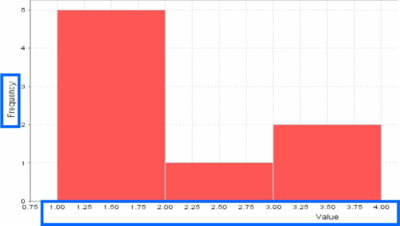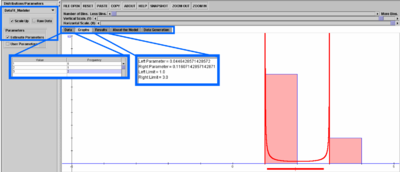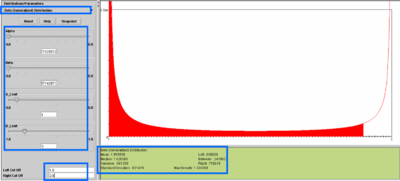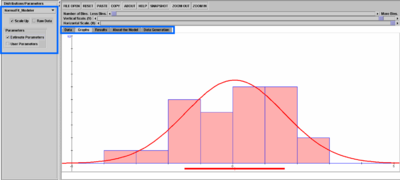# SOCR EduMaterials ModelerActivities NormalBetaModelFit

## SOCR Educational Materials - Activities - SOCR Normal and Beta Distribution Model Fit Activity

### Summary

This activity describes the process of SOCR model fitting in the case of using Normal or Beta distribution models. Model fitting is the process of determining the parameters for an analytical model in such a way that we obtain optimal parameter estimates according to some criterion. There are many strategies for parameter estimation. The differences between most of these are the underlying cost-functions and the optimization strategies applied to maximize/minimize the cost-function.

### Goals

The aims of this activity are to:

• motivate the need for (analytical) modeling of natural processes
• illustrate how to use the SOCR Modeler to fit models to real data
• present applications of model fitting

### Background & Motivation

Suppose we are given the sequence of numbers {1, 2, 3, 4, 5, 6, 7, 8, 9, 10} and asked to find the best (Continuous) Uniform Distribution that fits that data. In this case, there are two parameters that need to be estimated - the minimum (m) and the maximum (M) of the data. These parameters determine exactly the support (domain) of the continuous distribution and we can explicitly write the density for the (best fit) continuous uniform distribution as:

$$f(x) = {{1}\over{M-m}}$$, for $$m \le x \le M$$ and $$f(x)=0$$, for $$x \notin [m:M]$$.

Having this model distribution, we can use its analytical form, $$f(x)$$, to compute probabilities of events, critical functional values and, in general, do inference on the native process without acquiring additional data. Hence, a good strategy for model fitting is extremely useful in data analysis and statistical inference. Of course, any inference based on models is only going to be as good as the data and the optimization strategy used to generate the model.

Let's look at another motivational example. This time, suppose we have recorded the following (sample) measurements from some process {1.2, 1.4, 1.7, 3.4, 1.5, 1.1, 1.7, 3.5, 2.5}. Taking bin-size of 1, we can easily calculate the frequency histogram for this sample, {6, 1, 2}, as there are 6 observations in the interval [1:2), 1 measurement in the interval [2:3) and 2 measurements in the interval [3:4).We can now ask about the best Beta distribution model fit to the histogram of the data!

Most of the time when we study natural processes using probability distributions, it makes sense to fit distribution models to the frequency histogram of a sample, not the actual sample. This is because our general goals are to model the behavior of the native process, understand its distribution and quantify likelihoods of various events of interest (e.g., in terms of the example above, we may be interested in the probability of observing an outcome in the interval [1.50:2.15) or the chance that an observation exceeds 2.8).

### Exercises

#### Exercise 1

Let's first solve the challenge we presented in the background section, where we calculated the frequency histogram for a sample to be {6, 1, 2}. Go to the SOCR Modeler and click on the Data tab. Paste in the two columns of data. Column 1 {1, 2, 3} - these are the ranges of the sample values and correspond to measurements in the intervals [1:2), [2:3) and [3:4). The second column represents the actual frequency counts of measurements within each of these 3 histogram bins - these are the values {6, 1, 2}. Now press the Graphs tab. You should see an image like the one below. Then choose Beta_Fit_Modeler from the drop-down list of models in the top-left and click the estimate parameters check-box, also on the top-left. The graph now shows you the best Beta distribution model fit to the frequency histogram {6, 1, 2}. Click the Results tab to see the actual estimates of the two parameters of the corresponding Beta distribution (Left Parameter = 0.0446428571428572; Right Parameter = 0.11607142857142871; Left Limit = 1.0; Right Limit = 3.0).You can also see how the (general) Beta distribution degenerates to this shape by going to SOCR Distributions, selecting the (Generalized) Beta Distribution from the top-left and setting the 4 parameters to the 4 values we computed above. Notice how the shape of the Beta distribution changes with each change of the parameters. This is also a good demonstration of why we did the distribution model fitting to the frequency histogram in the first place - precisely to obtain an analytic model for studying the general process without acquiring mode data. Notice how we can compute the odds (probability) of any event of interest, once we have an analytical model for the distribution of the process. For example, this figure depicts the probabilities that a random observation from this process exceeds 2.8 (the right limit). This probability is computed to be 0.756#### Exercise 2

Go to the SOCR Modeler and select the Graphs tab and click the "Scale Up" check-box. Then select Normal_Model_Fit from the drop-down list of models and begin clicking on the graph panel. The latter allows you to construct manually a histogram of interest. Notice that these are not random measurements, but rather frequency counts that you are manually constructing the histogram of. Try to make the histogram bins form a unimodal, bell-shaped and symmetric graph. Observe that as you click, new histogram bins will appear and the model fit will update. Now click the Estimate Parameters check-box on the top-left and see the best-fit Normal curve appear superimposed on the manually constructed histogram. Under the Results tab you can find the maximum likelihood estimates for the mean and the standard deviation for the best Normal distribution fit to this specific frequency histogram.